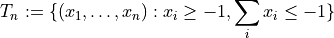# sfepy.discrete.simplex_cubature module¶

Generate simplex quadrature points. Code taken and adapted from pytools/hedge by Andreas Kloeckner.

sfepy.discrete.simplex_cubature.factorial(n)[source]
sfepy.discrete.simplex_cubature.generate_decreasing_nonnegative_tuples_summing_to(n, length, min=0, max=None)[source]
sfepy.discrete.simplex_cubature.generate_permutations(original)[source]

Generate all permutations of the list original’.

sfepy.discrete.simplex_cubature.generate_unique_permutations(original)[source]

Generate all unique permutations of the list original’.

sfepy.discrete.simplex_cubature.get_simplex_cubature(order, dimension)[source]

Cubature on an M{n}-simplex.

cf. A. Grundmann and H.M. Moeller, Invariant integration formulas for the n-simplex by combinatorial methods, SIAM J. Numer. Anal. 15 (1978), 282–290.

This cubature rule has both negative and positive weights. It is exact for polynomials up to order, whereis given as order. The integration domain is the unit simplexsfepy.discrete.simplex_cubature.wandering_element(length, wanderer=1, landscape=0)[source]